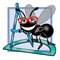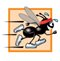# Recursion vs. Iteration

In the preceding sections, we studied methods factorial and fibonacci, which can easily be implemented either recursively or iteratively. In this section, we compare the two approaches and discuss why the programmer might choose one approach over the other in a particular situation.

Both iteration and recursion are based on a control statement: Iteration uses a repetition statement (e.g., for, while or do...while), whereas recursion uses a selection statement (e.g., if, if...else or switch). Both iteration and recursion involve repetition: Iteration explicitly uses a repetition statement, whereas recursion achieves repetition through repeated method calls. Iteration and recursion each involve a termination test: Iteration terminates when the loop-continuation condition fails, whereas recursion terminates when a base case is reached. Iteration with counter-controlled repetition and recursion each gradually approach termination: Iteration keeps modifying a counter until the counter assumes a value that makes the loop-continuation condition fail, whereas recursion keeps producing simpler versions of the original problem until the base case is reached. Both iteration and recursion can occur infinitely: An infinite loop occurs with iteration if the loop-continuation test never becomes false, whereas infinite recursion occurs if the recursion step does not reduce the problem each time in a manner that converges on the base case, or if the base case is not tested.

To illustrate the differences between iteration and recursion, let us examine an iterative solution to the factorial problem (Fig. 15.10Fig. 15.11). Note that a repetition statement is used (lines 1213 of Fig. 15.10) rather than the selection statement of the recursive solution (lines 912 of Fig. 15.3). Note that both solutions use a termination test. In the recursive solution, line 9 tests for the base case. In the iterative solution, line 12 tests the loop-continuation conditionif the test fails, the loop terminates. Finally, note that instead of producing simpler versions of the original problem, the iterative solution uses a counter that is modified until the loop-continuation condition becomes false.

Figure 15.10. Iterative factorial solution.

 ``` 1 // Fig. 15.10: FactorialCalculator.java 2 // Iterative factorial method. 3 4 public class FactorialCalculator 5 { 6 // recursive declaration of method factorial 7 public long factorial( long number ) 8 { 9 long result = 1; 10 11 // iterative declaration of method factorial 12 for ( long i = number; i >= 1; i-- ) 13 result *= i; 14 15 return result; 16 } // end method factorial 17 18 // output factorials for values 0-10 19 public void displayFactorials() 20 { 21 // calculate the factorials of 0 through 10 22 for ( int counter = 0; counter <= 10; counter++ ) 23 System.out.printf( "%d! = %d ", counter, factorial( counter ) ); 24 } // end method displayFactorials 25 } // end class FactorialCalculator ```

Figure 15.11. Testing the iterative factorial solution.

(This item is displayed on page 756 in the print version)

``` 1 // Fig. 15.11: FactorialTest.java
2 // Testing the iterative factorial method.
3
4 public class FactorialTest
5 {
6 // calculate factorials of 0-10
7 public static void main( String args[] )
8 {
9 FactorialCalculator factorialCalculator = new FactorialCalculator();
10 factorialCalculator.displayFactorials();
11 } // end main
12 } // end class FactorialTest
```

 ```0! = 1 1! = 1 2! = 2 3! = 6 4! = 24 5! = 120 6! = 720 7! = 5040 8! = 40320 9! = 362880 10! = 3628800 ```

Recursion has many negatives. It repeatedly invokes the mechanism, and consequently the overhead, of method calls. This repetition can be expensive in terms of both processor time and memory space. Each recursive call causes another copy of the method (actually, only the method's variables, stored in the activation record) to be createdthis set of copies can consume considerable memory space. Since iteration occurs within a method, repeated method calls and extra memory assignment are avoided. So why choose recursion?

Software Engineering Observation 15.1Any problem that can be solved recursively can also be solved iteratively (nonrecursively). A recursive approach is normally preferred over an iterative approach when the recursive approach more naturally mirrors the problem and results in a program that is easier to understand and debug. A recursive approach can often be implemented with fewer lines of code. Another reason to choose a recursive approach is that an iterative one might not be apparent.

Performance Tip 15.2Avoid using recursion in situations requiring high performance. Recursive calls take time and consume additional memory.

Common Programming Error 15.2Accidentally having a nonrecursive method call itself either directly or indirectly through another method can cause infinite recursion.Java How to Program (6th Edition) (How to Program (Deitel))
ISBN: 0131483986
EAN: 2147483647
Year: 2003
Pages: 615